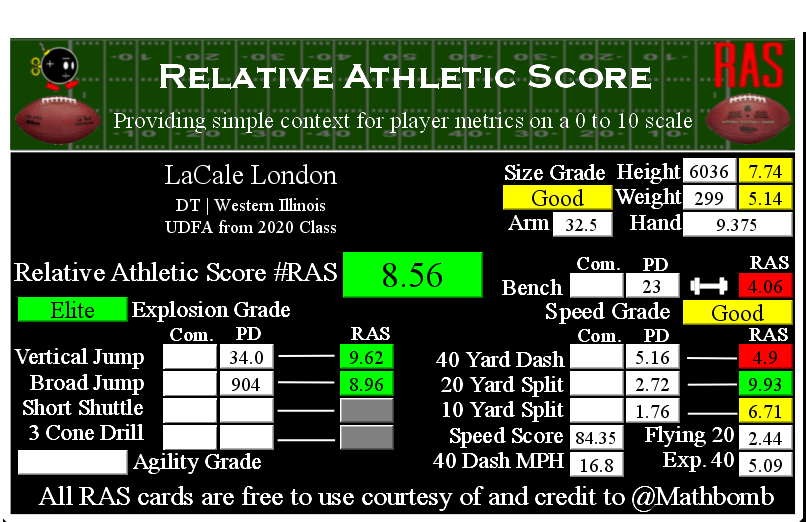# LaCale London RAS### LaCale London RASLaCale London went undrafted for the NFL out of Western Illinois in 2020.

He recorded a Relative Athletic Score of 8.56, out of a possible 10.0. RAS is a composite metric on a 0 to 10 scale based on the average of all of the percentile for each of the metrics the player completed either at the Combine or pro day.

He had a recorded height of 6036 that season, recorded as XYYZ where X is feet, YY is inches, and Z is eighths of an inch. That correlates to 6 feet, 3 and 6/8 of an inch or 75.75 inches, or 192.405 centimeters. This correlates to a 7.74 score out of 10.0.

He recorded a weight of 299 in pounds, which is approximately 135 kilograms. This correlates to a 5.14 score out of 10.0.

Based on his weight, he has a projected 40 yard dash time of 5.09. This is calculated by taking 0.00554 multiplied by his weight and then adding 3.433.

This player did not record any measurements at the NFL Combine that we were able to find.

At his pro day, he recorded a 40 yard dash of 5.16 seconds. This correlates to a 4.9 score out of 10.0.

The time traveled between the 20 and 40 yard lines is known as the Flying Twenty. As the distance is also known, we can calculate the player’s speed over that distance. The time he traveled the last twenty yards at his pro day was 2.44 seconds. Over 20 yards, we can calculate his speed in yards per second to 8.2. Taking into account the distance in feet (60 feet), we can calculate his speed in feet per second to 24.59. Breaking it down further, we can calculate his speed in inches per second to 295.08. Knowing the feet per second of 24.59, we can calculate the approximate miles per hour by multiplying that value by 0.681818 to give us a calculated MPH of 16.8 in the last 20 yards of his run.

At his pro day, he recorded a 20 yard split of 2.72 seconds. This correlates to a 9.93 score out of 10.0.

We can calculate the speed traveled over the second ten yards of the 40 yard dash easily, as the distance and time are both known. The time he traveled the second ten yards at his pro day was 0.96 seconds. Over 10 yards, we can calculate his speed in yards per second to 10.42. Taking into account the distance in feet (30 feet), we can calculate his speed in feet per second to 31.25. Breaking it down further, we can calculate his speed in inches per second to 375.0. Knowing the feet per second of 31.25, we can calculate the approximate miles per hour by multiplying that value by 0.681818 to give us a calculated MPH of 21.3 in the second ten yards of his run.

At his pro day, he recorded a 10 yard split of 1.76 seconds. This correlates to a 6.71 score out of 10.0.

The time he traveled the first ten yards at his pro day was 1.76 seconds. Over 10 yards, we can calculate his speed in yards per second to 6.0. Taking into account the distance in feet (30 feet), we can calculate his speed in feet per second to 17.0. Breaking it down further, we can calculate his speed in inches per second to 205.0. Knowing the feet per second of 17.0, we can calculate the approximate miles per hour by multiplying that value by 0.681818 to give us a calculated MPH of 11.6 in the first ten yards of his run.

At his pro day, he recorded a bench press of 23 repetitions of 225 pounds. This correlates to a 4.06 score out of 10.0.

At his pro day, he recorded a vertical jump of 34.0 seconds. This correlates to a 9.62 score out of 10.0.

At his pro day, he recorded a broad jump of 904, which is recorded as FII or FFII where F is feet and I is inches. This correlates to a 8.96 score out of 10.0.

This site uses Akismet to reduce spam. Learn how your comment data is processed.